# 22 Results

View
Selected filters:
• Pythagorean Theorem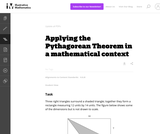Unrestricted Use
CC BY
Rating

This task requires students to apply the Pythagorean Theorem.

Subject:
Math
Material Type:
Activity/Lab
Provider:
Illustrative Mathematics
Provider Set:
Illustrative Mathematics
Author:
Illustrative Mathematics
05/01/2012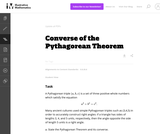Unrestricted Use
CC BY
Rating

This task is for instruction purposes. Part (b) is subtle and the solution presented here uses a "dynamic" view of triangles with two side lengths fixed. This helps pave the way toward what students will see later in trigonometry but some guidance will likely be needed in order to get students started on this path.

Subject:
Math
Material Type:
Activity/Lab
Provider:
Illustrative Mathematics
Provider Set:
Illustrative Mathematics
Author:
Illustrative Mathematics
08/17/2012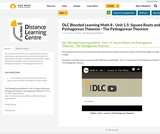Conditional Remix & Share Permitted
CC BY-NC-SA
Rating

The purpose of this lesson is to use what has been learned about squares and square roots to find the side lengths of a right triangle.

Included is a YouTube video to support Grade 8 Blended Learning Math - Unit 1.5: Square Roots and Pythagorean Theorem - The Pythagorean Theorem.

Subject:
Math
Material Type:
Activity/Lab
Homework/Assignment
Lesson
Provider:
Sun West Distance Learning Centre (DLC)
Author:
Sun West School Division
06/14/2019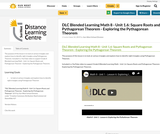Conditional Remix & Share Permitted
CC BY-NC-SA
Rating

The purpose of this lesson is to look at various triangles and explore how to identify right triangles using Pythagorean Theorem.

Included is a YouTube video to support Grade 8 Blended Learning Math - Unit 1.6: Square Roots and Pythagorean Theorem - Exploring the Pythagorean Theorem.

Subject:
Math
Material Type:
Activity/Lab
Homework/Assignment
Lesson
Provider:
Sun West Distance Learning Centre (DLC)
Author:
Sun West School Division
06/14/2019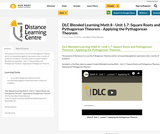Conditional Remix & Share Permitted
CC BY-NC-SA
Rating

The purpose of this lesson is to use the Pythagorean Theorem and its corresponding algebraic expression solve a variety of problems.

Included is a YouTube video to support Grade 8 Blended Learning Math - Unit 1.7: Square Roots and Pythagorean Theorem - Applying the Pythagorean Theorem.

Subject:
Math
Material Type:
Activity/Lab
Homework/Assignment
Lesson
Provider:
Sun West Distance Learning Centre (DLC)
Author:
Sun West School Division
06/14/2019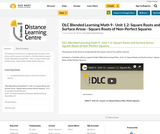Conditional Remix & Share Permitted
CC BY-NC-SA
Rating

The purpose of this lesson is to approximate the square roots of non-perfect squares.

Included is a YouTube video to support Grade 9 Blended Learning Math - Unit 1.2: Square Roots and Surface Areas - Square Roots of Non-Perfect Squares.

Subject:
Math
Material Type:
Activity/Lab
Homework/Assignment
Lesson
Provider:
Sun West Distance Learning Centre (DLC)
Author:
Sun West School Division
06/18/2019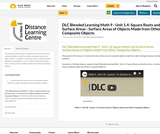Conditional Remix & Share Permitted
CC BY-NC-SA
Rating

The purpose of this lesson is to determine the surface area of composite objects made from right rectangular prisms and right cylinders.

Included is a YouTube video to support Grade 9 Blended Learning Math - Unit 1.4: Square Roots and Surface Areas - Surface Areas of Objects Made from Other Composite Objects.

Subject:
Math
Material Type:
Activity/Lab
Homework/Assignment
Lesson
Provider:
Sun West Distance Learning Centre (DLC)
Author:
Sun West School Division
06/18/2019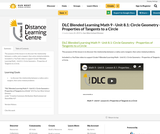Conditional Remix & Share Permitted
CC BY-NC-SA
Rating

The purpose of this lesson is to discover the relationship between a radius and a tangent, then solve related problems.

Included is a YouTube video to support Grade 9 Blended Learning Math - Unit 8.1: Circle Geometry - Properties of Tangents to a Circle.

Subject:
Math
Material Type:
Activity/Lab
Homework/Assignment
Lesson
Provider:
Sun West Distance Learning Centre (DLC)
Author:
Sun West School Division
06/24/2019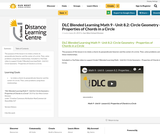Conditional Remix & Share Permitted
CC BY-NC-SA
Rating

The purpose of this lesson is to relate a chord, its perpendicular bisector and the center of a circle. Then, solve problems using these relationships.

Included is a YouTube video to support Grade 9 Blended Learning Math - Unit 8.2: Circle Geometry - Properties of Chords in a Circle.

Subject:
Math
Material Type:
Activity/Lab
Homework/Assignment
Lesson
Provider:
Sun West Distance Learning Centre (DLC)
Author:
Sun West School Division
06/24/2019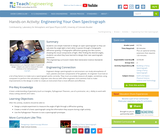Read the Fine Print
Educational Use
Rating

Students use simple materials to design an open spectrograph so they can calculate the angle light is bent when it passes through a holographic diffraction grating. A holographic diffraction grating acts like a prism, showing the visual components of light. After finding the desired angles, students use what they have learned to design their own spectrograph enclosure.

Subject:
Physics
Material Type:
Activity/Lab
Provider:
TeachEngineering
Provider Set:
TeachEngineering
10/14/2015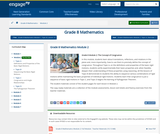Conditional Remix & Share Permitted
CC BY-NC-SA
Rating

In this module, students learn about translations, reflections, and rotations in the plane and, more importantly, how to use them to precisely define the concept of congruence. Throughout Topic A, on the definitions and properties of the basic rigid motions, students verify experimentally their basic properties and, when feasible, deepen their understanding of these properties using reasoning. All the lessons of Topic B demonstrate to students the ability to sequence various combinations of rigid motions while maintaining the basic properties of individual rigid motions. Students learn that congruence is just a sequence of basic rigid motions in Topic C, and Topic D begins the learning of Pythagorean Theorem.

Subject:
Math
Material Type:
Module
Provider:
New York State Education Department
Provider Set:
EngageNY
09/21/2013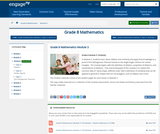Conditional Remix & Share Permitted
CC BY-NC-SA
Rating

In Module 3, students learn about dilation and similarity and apply that knowledge to a proof of the Pythagorean Theorem based on the Angle-Angle criterion for similar triangles.  The module begins with the definition of dilation, properties of dilations, and compositions of dilations.  One overarching goal of this module is to replace the common idea of same shape, different sizes with a definition of similarity that can be applied to geometric shapes that are not polygons, such as ellipses and circles.

Subject:
Math
Material Type:
Module
Provider:
New York State Education Department
Provider Set:
EngageNY
10/17/2013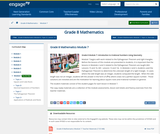Conditional Remix & Share Permitted
CC BY-NC-SA
Rating

Module 7 begins with work related to the Pythagorean Theorem and right triangles.  Before the lessons of this module are presented to students, it is important that the lessons in Modules 2 and 3 related to the Pythagorean Theorem are taught (M2:  Lessons 15 and 16, M3:  Lessons 13 and 14).  In Modules 2 and 3, students used the Pythagorean Theorem to determine the unknown length of a right triangle.  In cases where the side length was an integer, students computed the length.  When the side length was not an integer, students left the answer in the form of x2=c, where c was not a perfect square number.  Those solutions are revisited and are the motivation for learning about square roots and irrational numbers in general.

Subject:
Math
Material Type:
Module
Provider:
New York State Education Department
Provider Set:
EngageNY
02/02/2014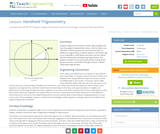Read the Fine Print
Educational Use
Rating

Students explore the concept of similar right triangles and how they apply to trigonometric ratios. Use this lesson as a refresher of what trig ratios are and how they work. In addition to trigonometry, students explore a clinometer app on an Android® or iOS® device and how it can be used to test the mathematics underpinning trigonometry. This prepares student for the associated activity, during which groups each put a clinometer through its paces to better understand trigonometry.

Subject:
Math
Material Type:
Lesson
Provider:
TeachEngineering
Provider Set:
TeachEngineering
Author:
Scott Burns
09/18/2014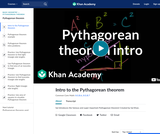Conditional Remix & Share Permitted
CC BY-NC-SA
Rating

Sal introduces the famous and super important Pythagorean theorem! This lesson includes an introduction video followed by examples and practice problems involving finding the hypotenuse, finding a side, finding area of an isosceles triangle, and determining if a triangle is a right triangle. It is followed by a unit on Pythagorean theorem applications.

Subject:
Math
Material Type:
Homework/Assignment
Lesson
Provider:
Author:
Salman Khan
10/10/2018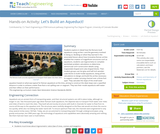Read the Fine Print
Educational Use
Rating

Students explore in detail how the Romans built aqueducts using arches—and the geometry involved in doing so. Building on what they learned in the associated lesson about how innovative Roman arches enabled the creation of magnificent structures such as aqueducts, students use trigonometry to complete worksheet problem calculations to determine semicircular arch construction details using trapezoidal-shaped and cube-shaped blocks. Then student groups use hot glue and half-inch wooden cube blocks to build model aqueducts, doing all the calculations to design and build the arches necessary to support a water-carrying channel over a three-foot span. They calculate the slope of the small-sized aqueduct based on what was typical for Roman aqueducts at the time, aiming to construct the ideal slope over a specified distance in order to achieve a water flow that is not spilling over or stagnant. They test their model aqueducts with water and then reflect on their performance.

Subject:
Math
Material Type:
Activity/Lab
Provider:
TeachEngineering
Author:
Lauchlin Blue
Malinda Zarske
Nathan Coyle
05/07/2018Rating

This amazing site from Alberta has interactive for all the strands.
These could be used by students or in small group instruction or as a whole class.

Subject:
Math
Material Type:
Activity/Lab
Author:
Learn Alberta
10/31/2018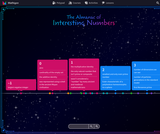Rating

Discover Mathigon, the Mathematical Playground. Learning mathematics has never been so interactive and fun!
Check out:
Polypad - Virtual manipulatives, dynamic geometry, graph plotting, data science and more: explore the ultimate mathematical playground!
Multiplication by Heart - These beautiful flash cards use spaced repetition to teach multiplication facts. Achieve fluency with just five minutes of practice per day!
Activities - A selection of our favourite mathematical puzzles and problems. Most are simple to understand, but the solutions require clever and unconventional thinking.
Factris - A fun game that teaches about simple arithmetic
Course Library - Mathigon's innovative courses cover everything from fractions and trigonometry to graph theory, cryptography, prime numbers and fractals.
Puzzles, Activities and Lesson Plans - Student Explorations/Activities for students to complete ; Fully developed lessons plans ; Ready to play puzzles and games ; Teaching ideas using Polypad to explore new ideas; Tutorials - Learn how to use Polypad
Almanac of Interesting Numbers
And much more!

Subject:
Math
Material Type:
Activity/Lab
Game
Homework/Assignment
Simulation
Author:
Mathigon
09/15/2022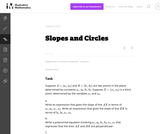Unrestricted Use
CC BY
Rating

The purpose of this task is to lead students through an algebraic approach to a well-known result from classical geometry, namely, that a point X is on the circle of diameter AB whenever _AXB is a right angle.

Subject:
Math
Material Type:
Activity/Lab
Provider:
Illustrative Mathematics
Provider Set:
Illustrative Mathematics
Author:
Illustrative Mathematics
05/01/2012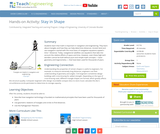Read the Fine Print
Educational Use
Rating

Students learn that math is important in navigation and engineering. They learn about triangles and how they can help determine distances. Ancient land and sea navigators started with the most basic of navigation equations (speed x time = distance). Today, navigational satellites use equations that take into account the relative effects of space and time. However, even these high-tech wonders cannot be built without pure and simple math concepts â basic geometry and trigonometry â that have been used for thousands of years.

Subject:
Science
Material Type:
Activity/Lab
Provider:
TeachEngineering
Provider Set:
TeachEngineering
Author:
Janet Yowell
Jeff White
Malinda Schaefer Zarske
Matt Lippis